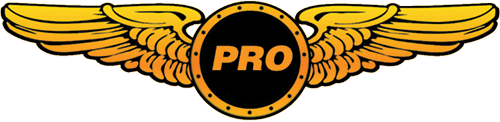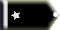TechyJay Trainee

Is it possible for anyone to upload the Grumman Goose plane for FSX. It comes with the deluxe edition, but I do not have that version. The store doesn't let me exchange, and the only other way to get this plane is to download a slightly modified version. if anyone could upload this plane it would be greatly apreciated.The grumman goose is my favorite plane ever!
whats yours?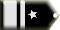Tailhook Chief Captain

The Goose is about 72MB in size. To upload it on a slow connection is a big ask. At this stage I don't even know whether it would work.

You could try to get Lynn and Bill Lyons' fs9 Goose to work in FSX.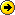http://library.avsim.net/search.php?SearchTerm=grumman+goose&CatID=fs2004ac&Go=Search

This is a rather big package and includes many things you won't need. As it comes with an .exe file, you will be in trouble straight away if you make use of it.
I can only suggest the following:

Create a temporary folder. Run the .exe and make sure you target the temporary folder you just created - this is very important.

Now find the folder aircraft. Open it and find the folder G21.

This is the folder that should go into your SimObjects / Airplanes Directory.

To be on the safe side, exchange the existing panel with one from FSX such as the Beech Kingair or Beech Baron. This should ensure that you won't have problems with outdated gauges.

In the folder cc9g21f2 you will find the folder effects. You can try and move the 20 fx_xxx files into your FSX Effects directory - they shouldn't cause any harm.
As a security measure though, I'd make sure to remember exactly which effects I've added so I could delete them if the need arises.

The above is my theory, there is a chance it might work and if you follow the common sense guidelines, you shouldn't cause any harm to your FSX installation.TechyJay Trainee

Ok i'll give it a shot, but last time i tryed to get this FS9 plane onto FSX it didn't land on water properly, it just sank... so uh... it was kind of useless. If anyone could upload one here that works for sure that would be excellent but i'll post again when I try the one in the above link.TechyJay Trainee

I have just realized that the Grumman_Goose_G21A folder s already in my aircraft folder. It is not in the game though. FSX must somehow be disabling it. Does anyone know how to enable it, or change some part of the code that will make it show up? Any ideas?Tailhook Chief Captain

TechyJay wrote:

I have just realized that the Grumman_Goose_G21A folder s already in my aircraft folder. It is not in the game though. FSX must somehow be disabling it. Does anyone know how to enable it, or change some part of the code that will make it show up? Any ideas?

It is the folder G21 that should be in your Airplanes Directory as I've already mentioned.

...find the folder G21.

This is the folder that should go into your SimObjects / Airplanes Directory.

Now the aircraft should show up under 'Grumman' (that's the manufacturer).

I don't know whether you've made changes to your aircraft.cfg without making a backup. This is the original one in case you need it:

[fltsim.0]
title=Grumman Goose G21a Cutters
sim=g21
model=
panel=
sound=
texture=
panel_alias =
sound_alias =
kb_checklists=goose_check
kb_reference=goose_ref
visual_damage=1
atc_id=NC327
prop_anim_ratio=0.45
ui_manufacturer=Grumman
ui_type= Cutters Goose
ui_variation=
description=Grumman Goose N327 as it appeared in the flight scenes in Tales of the Gold Monkey.
atc_heavy=0
atc_airline=
atc_flight_number=

[General]
atc_type=Grumman
atc_model=G21
editable=1
performance=See Kneeboard Reference

[fltsim.1]
title=Grumman Goose JRF-2
sim=g21
model=
panel=
sound=
texture=1
panel_alias =
sound_alias =
kb_checklists=goosej_check
kb_reference=goosej_ref
visual_damage=1
atc_id=1050
prop_anim_ratio=0.45
ui_manufacturer=Grumman
ui_type= Goose JRF-2
ui_variation=
description=Grumman JRF-2 in WW2 Coastal Patrol colors.
atc_heavy=0
atc_airline=navy
atc_flight_number=1050

[fltsim.2]
title=Grumman Goose G21a Avalon Air Transport
sim=g21
model=
panel=
sound=
texture=2
panel_alias =
sound_alias =
kb_checklists=Goosea_check
kb_reference=Goosea_ref
visual_damage=1
atc_id=N327
prop_anim_ratio=0.45
ui_manufacturer=Grumman
ui_type= Goose Avalon Air Transport
ui_variation=
description=Grumman Goose N327 as it appeared while used by Avalon Air Transport to ferry passengers to Catalina Island.
atc_heavy=0
atc_airline=
atc_flight_number=

[fltsim.3]
title=Grumman Goose G21a Southeast Skyways
sim=g21
model=
panel=
sound=
texture=3
panel_alias =
sound_alias =
kb_checklists=Gooses_check
kb_reference=Gooses_ref
visual_damage=1
atc_id=N327
prop_anim_ratio=0.45
ui_manufacturer=Grumman
ui_type= Goose Southeast Skyways
ui_variation=
description=Grumman Goose N327 as it appeared while used by Southeast Skyways operating out of Juneau Alaska.
atc_heavy=0
atc_airline=
atc_flight_number=

[fltsim.4]
title=Grumman Goose G21a Chrysler Air
sim=g21
model=
panel=
sound=
texture=4
panel_alias =
sound_alias =
kb_checklists=Goosec_check
kb_reference=Goosec_ref
visual_damage=1
atc_id=N327
prop_anim_ratio=0.45
ui_manufacturer=Grumman
ui_type= Goose Chrylsler Air
ui_variation=
description=Grumman Goose N327 as it appeared while used by Chrysler Air in Seattle Washington
atc_heavy=0
atc_airline=
atc_flight_number=

[fltsim.5]
title=Grumman Goose JRF-2 NAVY2
sim=g21
model=
panel=
sound=
texture=5
panel_alias =
sound_alias =
kb_checklists=goosej_check
kb_reference=goosej_ref
visual_damage=1
atc_id=1050
prop_anim_ratio=0.45
ui_manufacturer=Grumman
ui_type= Goose JRF-2 Navy2
ui_variation=
description=Grumman JRF-2 in WW2 NAVY Colors
atc_heavy=0
atc_airline=navy
atc_flight_number=1050

[fltsim.6]
title=Grumman Goose JRF-2 Peruvian Army Air Force
sim=g21
model=
panel=
sound=
texture=6
panel_alias =
sound_alias =
kb_checklists=goosej_check
kb_reference=goosej_ref
visual_damage=1
atc_id=1050
prop_anim_ratio=0.45
ui_manufacturer=Grumman
ui_type= Goose JRF-2 Peruvian Army Air Force
ui_variation=
description=Grumman JRF-2 in WW2 Peruvian Colors
atc_heavy=0
atc_airline=army
atc_flight_number=1051

[fltsim.7]
title=Grumman Goose G21a Cutters with Sarah
sim=g21
model=1
panel=
sound=
texture=
panel_alias =
sound_alias =
kb_checklists=goose_check
kb_reference=goose_ref
visual_damage=1
atc_id=NC327
prop_anim_ratio=0.45
ui_manufacturer=Grumman
ui_type= Cutters Goose Sarah Copilot
ui_variation=
description=Grumman Goose N327 as it appeared in the flight scenes in Tales of the Gold Monkey.

[forcefeedback]
ground_bumps_magnitude1=2600 ; 0 - 10000
ground_bumps_angle1=8900 ; 0 - 35999 degrees
ground_bumps_intercept1=5.0
ground_bumps_slope1=0.6
ground_bumps_magnitude2=1200 ; 0 - 10000
ground_bumps_angle2=9100 ; 0 - 35999 degrees
ground_bumps_intercept2=0.085
ground_bumps_slope2=0.65
crash_magnitude1=10000 ; 0 - 10000
crash_direction1=01000 ; 0 - 35999 degrees
crash_magnitude2=10000 ; 0 - 10000
crash_direction2=9000 ; 0 - 35999 degrees
crash_period2=75000 ; in microseconds
crash_duration2=3500000 ; in microseconds

[flight_tuning]
cruise_lift_scalar=0.34
parasite_drag_scalar=0.22
induced_drag_scalar=1.0
elevator_effectiveness=1.3
aileron_effectiveness=1.1
rudder_effectiveness=2.3
pitch_stability=1.0
roll_stability=1.0
yaw_stability=1.0
elevator_trim_effectiveness=0.5
aileron_trim_effectiveness=1.0
rudder_trim_effectiveness=1.0

[WEIGHT_AND_BALANCE]
reference_datum_position=0.000000,0.000000,0.000000
empty_weight_CG_position=0.000000,0.000000,0.000000
max_number_of_stations = 50
station_load.0 = "170, -4.0, -1.5, 0.0, Pilot" // Weight (lbs), longitudinal, lateral, vertical positions from datum (feet)
station_load.1 = "190, -4.0, 1.5, 0.0, Copilot" // Weight (lbs), longitudinal, lateral, vertical positions from datum (feet)
station_load.2 = "10, 0.0, 0.0, 0.0, Rear Passenger" // Weight (lbs), longitudinal, lateral, vertical positions from datum (feet)
station_load.3 = "0, 0.0, 0.0, 0.0, Rear Passenger" // Weight (lbs), longitudinal, lateral, vertical positions from datum station_load.4 = "0, 0.0, 0.0, 0.0, Rear Passenger" // Weight (lbs), longitudinal, lateral, vertical positions from datum station_load.5 = "0, 0.0, 0.0, 0.0, Rear Passenger" // Weight (lbs), longitudinal, lateral, vertical positions from datum station_load.6 = "0, 0.0, 0.0, 0.0, Rear Passenger" // Weight (lbs), longitudinal, lateral, vertical positions from datum station_load.7 = "0, 0.0, 0.0, 0.0, Rear Passenger" // Weight (lbs), longitudinal, lateral, vertical positions from datum (feet)
station_load.4 = "0, 0.0, 0.0, 0.0, Rear Passenger"
station_load.5 = "0, 0.0, 0.0, 0.0, Rear Passenger"
station_load.6 = "0, 0.0, 0.0, 0.0, Rear Passenger"
station_load.7 = "0, 0.0, 0.0, 0.0, Cargo"
station_load.8 = "30, 0.0, 0.0, 0.0, Baggage" // Weight (lbs), longitudinal, lateral, vertical positions from datum (feet)

empty_weight=4856.000000
empty_weight_roll_MOI=13273.000000
empty_weight_pitch_MOI=13335.000000
empty_weight_yaw_MOI=11655.000000
empty_weight_coupled_MOI=0.000000
max_gross_weight=8000.00000

[fuel]
LeftMain=0.000, 0.000, 0.000, 70.000, 0.000
RightMain=0.000, 0.000, 0.000, 70.000, 0.000
fuel_type=1.000000
number_of_tank_selectors=1
electric_pump=0

[airplane_geometry]
wing_area=375.000000
wing_span=49.500000
wing_root_chord=7.916667
wing_dihedral=0.998779
wing_incidence=2.499634
wing_twist=0.000000
oswald_efficiency_factor=1.070
wing_winglets_flag=0
wing_sweep=0.000000
wing_pos_apex_lon=-2.000000
wing_pos_apex_vert=0.000000
htail_area=32.000000
htail_span=12.250000
htail_pos_lon=-16.416667
htail_pos_vert=0.000000
htail_incidence=-0.697632
htail_sweep=0.000000
vtail_area=69.000000
vtail_span=6.140033
vtail_sweep=0.000000
vtail_pos_lon=-27.916667
vtail_pos_vert=1.500000
elevator_area=12.820000
aileron_area=18.070000
rudder_area=18.230000
elevator_up_limit=28.000447
elevator_down_limit=20.998903
aileron_up_limit=16.999658
aileron_down_limit=16.999658
rudder_limit=19.996227
elevator_trim_limit=19.996227
spoiler_limit=20.000000
spoilerons_available=0
aileron_to_spoileron_gain=0.000000
min_ailerons_for_spoilerons=0.000000
min_flaps_for_spoilerons=0.000000

//0 Class
//1 Longitudinal Position (feet)
//2 Lateral Position (feet)
//3 Vertical Position (feet)
//4 Impact Damage Threshold (Feet Per Minute)
//5 Brake Map (0=None, 1=Left, 2=Right)
//7 Steer Angle (degrees)
//8 Static Compression (feet) (0 if rigid)
//9 Max/Static Compression Ratio
//10 Damping Ratio (0=Undamped, 1=Critically Damped)
//11 Extension Time (seconds)
//12 Retraction Time (seconds)
//13 Sound Type

[contact_points]

point.0 =1, -16.80, 0.00, -1.80, 3200, 0, 0.70,70.0, 0.30, 2.5, 0.90, 4.5, 4.5, 0, 0, 190
point.1 =1, 1.40, -3.91, -3.90, 2200, 1, 1.40, 0.0, 0.50, 2.5, 0.85, 0.0, 0.0, 2, 0, 0
point.2 =1, 1.40, 3.91, -3.90, 2200, 2, 1.40, 0.0, 0.50, 2.5, 0.85, 5.5, 5.5, 3, 0, 190
point.3 = 4, 4.00, -1.50, -1.410, 36000, 0, 0.00, 47.0, 0.85, 40.5, 1.25, 0.0, 0.0, 0, 0.0, 0.0
point.4 = 4, 4.00, 1.50, -1.410, 36000, 0, 0.00, 47.0, 0.85, 40.5, 1.25, 0.0, 0.0, 0, 0.0, 0.0
point.5 = 4, -4.35, -1.250, -1.310, 36000, 0, 0.00, 0.0, 0.85, 40.5, 3.25, 0.0, 0.0, 0, 0.0, 0.0
point.6 = 4, -4.35, 1.250, -1.310, 36000, 0, 0.00, 0.0, 0.85, 40.5, 3.25, 0.0, 0.0, 0, 0.0, 0.0
point.7 = 2, -3.35, -16.250, 0.200, 136000, 0, 0.00, 0.0, 0.0, 0.0, 0.0, 0.0, 0.0, 0, 0.0, 0.0
point.8 = 2, -3.35, 16.250, 0.200, 136000, 0, 0.00, 0.0, 0.0, 0.0, 0.0, 0.0, 0.0, 0, 0.0, 0.0
point.9 = 5, 0.90, 0.0, 7.300, 1600, 0, 0.00, 50.0, 2.0, 2.0, 2.00, 2.0, 2.0, 2.0,2.0, 2.0
point.10 = 4, -14.35, 0.0, 0.20, 136000,1, 1.40, 0.0, 0.50, 5.5, 0.85, 0.0, 0.0, 0, 0, 0

static_cg_height = 3.20 //(feet) altitude of CG when at rest (altitude when loaded on ground)
static_pitch = 6.50 //degrees (pitch when loaded on ground)
gear_system_type=0

[Flaps.0]
type=1
span-outboard=0.500000
extending-time=5.000000
system_type=0
flaps-position.0=0.000000,0.000000
flaps-position.1=7.000,0.000000
flaps-position.2=12.000,0.000000
flaps-position.3=17.000,0.000000
flaps-position.4=25.000,0.000000

lift_scalar = 1.0
drag_scalar = 1.0
//pitch_scalar= 0.0

[GeneralEngineData]
engine_type=0
Engine.0=0.500000,-6.000000,1.000000
Engine.1=0.500000, 6.000000,1.000000
fuel_flow_scalar=1.000000
min_throttle_limit=0.0000

[piston_engine]
cylinder_displacement=83.000000
compression_ratio=7.000000
number_of_cylinders=9
max_rated_rpm=2300.000000
max_rated_hp=350.000000
fuel_metering_type=1
cooling_type=0
normalized_starter_torque=0.0775000
turbocharged=0
max_design_mp=40.999999
min_design_mp=1.000000
critical_altitude=17000.000000
emergency_boost_type=2
emergency_boost_mp_offset=0.000000
emergency_boost_gain_offset=0.000000
fuel_air_auto_mixture=1
auto_ignition=0
max_rpm_mechanical_efficiency_scalar=1.000000
idle_rpm_mechanical_efficiency_scalar=1.000000
max_rpm_friction_scalar=1.000000
idle_rpm_friction_scalar=0.450000
power_scalar =1.00

[propeller]
propeller_type=0
propeller_diameter=7.0000
propeller_moi=25.400000
beta_max=60.000000
beta_min=7.000000
min_gov_rpm=1800.000000
prop_tc=0.010000
gear_reduction_ratio=1.20000
fixed_pitch_beta=0.000000
low_speed_theory_limit=80.000000
prop_sync_available=0
prop_deice_available=0
prop_feathering_available= 0 //Prop feathering available? 0=FALSE, 1=TRUE
prop_auto_feathering_available= 0 //Prop auto-feathering available? 0=FALSE, 1=TRUE
min_rpm_for_feather= 1100.0 //Minimum RPM for prop feathering
beta_feather= 89.5 //Feathering pitch angle (degrees)
power_absorbed_cf= 1.9 //Coefficient of friction for power absorbed by propeller in feather
defeathering_accumulators_available= 0 //Defeathering accumulators available? 0=FALSE, 1=TRUE
prop_reverse_available= 0 //Prop reverse available? 0=FALSE, 1=TRUE
minimum_on_ground_beta= 0.0 //Miminum pitch angle on ground, (degrees)
minimum_reverse_beta= 0.0 //Minimum pitch angle in reverse, (degrees)
feathering_switches = 0 //Switches which invoke feathering
thrust_scalar =1.40

[SMOKESYSTEM]
smoke.0=12.0, 0.0, -6.30, fx_goosefire

[EFFECTS]
wake=fx_wake
dirt=fx_dustrun
concrete=fx_sparksx
touchdown=fx_tchdwn_s, 1
startup=fx_engstrt_g21
water=fx_goose_spray

[keyboard_response]
elevator=300.000000,500.000000
aileron=150.000000,300.000000
rudder=200.000000,1000.000000

[Views]
eyepoint=-0.800000,-1.30000,4.100000

[exits]
number_of_exits = 1
exit_rate.0 = 0.4 ;Percent per second

[LIGHTS]
light.0=2, -0.0, -21.4, 6.10, fx_navredc
light.1=2, -0.0, 21.4, 6.10, fx_navgrec
light.2=2, -21.0, 0.00, 10.70, fx_navredc
light.3=4, -3.0, 0.00, 2.5, fx_vclight_goose
light.4=4, -10.0, 0.00, 4.0, fx_vclight_goose
xxlight.5=4, 2.2, 0.00,3.2, fx_vclight_goosef

// Radio Type = availiable, standby frequency, has glide slope
Audio.1 = 1
Com.1 = 1
Nav.1 = 1
Nav.2 = 1
Transponder.1 = 1

[electrical]
flap_motor = 0, 5 , 17.0
gear_motor = 0, 5 , 17.0
avionics_bus = 0, 5 , 17.0
avionics = 1, 5 , 17.0
pitot_heat = 0, 2 , 17.0
starter1 = 0, 20, 17.0
starter2 = 0, 20, 17.0
light_nav = 0, 5 , 17.0
light_beacon = 0, 5 , 17.0
light_landing = 0, 5 , 17.0
light_panel = 0, 5 , 17.0

[autopilot]
autopilot_available=1
flight_director_available=0
default_vertical_speed=700.000000
autothrottle_available=0
pitch_takeoff_ga=8.000000
max_pitch=10.000000
max_pitch_acceleration=1.000000
max_pitch_velocity_lo_alt=2.000000
max_pitch_velocity_hi_alt=1.500000
max_pitch_velocity_lo_alt_breakpoint=20000.000000
max_pitch_velocity_hi_alt_breakpoint=28000.000000
max_bank=25.000000
max_bank_acceleration=1.800000
max_bank_velocity=3.000000
max_throttle_rate=0.100000
nav_proportional_control=9.000000
nav_integrator_control=0.250000
nav_derivative_control=0.000000
nav_integrator_boundary=2.500000
nav_derivative_boundary=0.000000
gs_proportional_control=9.520000
gs_integrator_control=0.260000
gs_derivative_control=0.000000
gs_integrator_boundary=0.700000
gs_derivative_boundary=0.000000
yaw_damper_gain=0.000000

[direction_indicators]
direction_indicator.0=1,0

[attitude_indicators]
attitude_indicator.0=1

[turn_indicators]
turn_indicator.0=1,0

[Reference Speeds]
flaps_up_stall_speed=60.000000
full_flaps_stall_speed=47.600000
cruise_speed=167.0
max_indicated_speed=260.00000

[brakes]
parking_brake = 1 //Parking brake available
toe_brakes_scale = 0.7 //Brake scalar
differential_braking_scale = 1.0 //Delta on the amount of brake when the rudder pedals deflected

[gear_warning_system]
gear_warning_available=0
pct_throttle_limit=0.000000
flap_limit_power=0.000000
flap_limit_idle=0.000000

[hydraulic_system]
normal_pressure = 732.0 //PSI
electric_pumps = 0 //no electric pumps
engine_map = 1,1,0,0 //pumps on Eng1,2

[stall_warning]
type=0

[TailHook]
tailhook_length=4 ;(feet)
tailhook_position = -17.5, 0.0, -0.5 ;(feet) longitudinal, lateral, vertical positions from datum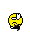TechyJay Trainee

as i had expected with the last FS9 plane i uploaded, it does not land in the water... it sinks... so there isn't a point of me having the FS9 one. I somehow need a way to get the Grumman Goose from FSX onto my game, i paid to register to this site, can noone find out how to get the FSX plane on it. Is it impossible?thanks for all of you help sofarmike20022 Guest

it say's all you need to do is add a panel folder so i added a panel folder and it worked but there are no gauges in the virtual cockpit can anyone help on how to add the gauges in the wright placeGuest

Hi: I did exactly the above and got the same results, no gauges on V/C therefore no A/P. Although all the gauges are in the panel folder including the rain effects and .cab. When loading the plane oone is forced to disable a lot of the gauges, you know the messaqge: they're no longer supported!
Does anybody know how the panel of the Goose look like in FSX deluxe?
What about a screeny?...guest Guest

Hy,can someone upload just the panel folder from the Grumman_Goose_G21A folder, in the simulator x deluxe edition?

With adding just this folder ,the plane works in the ordinary version of simulator too.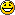mike20022 Guest

hi i found this website that has the full panel's for most of the plane's
it work's with the V/C all gauge's work

I downloaded the Grumman_Goose_G21A.exe Maule_M7_260C.exe and the Maule_M7_260C_Ski.exe unzip them put the panel's in the right folder
and the plane's work fullyAll times are GMT Page 1 of 1